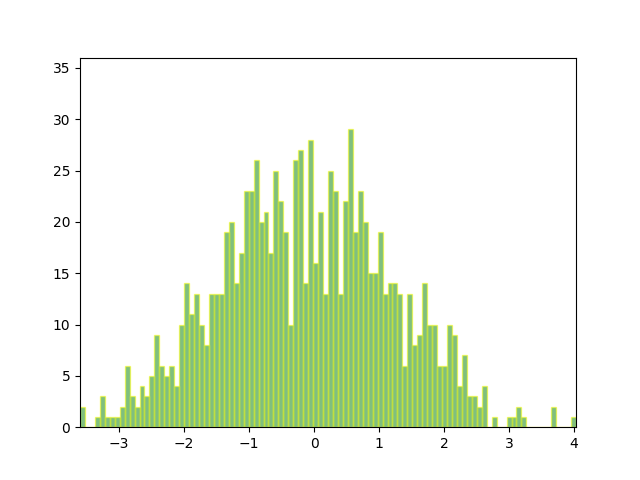Travis-CI:# Animated histogram¶

This example shows how to use a path patch to draw a bunch of rectangles for an animated histogram.```import numpy as np

import matplotlib.pyplot as plt
import matplotlib.patches as patches
import matplotlib.path as path
import matplotlib.animation as animation

fig, ax = plt.subplots()

# Fixing random state for reproducibility
np.random.seed(19680801)

# histogram our data with numpy
data = np.random.randn(1000)
n, bins = np.histogram(data, 100)

# get the corners of the rectangles for the histogram
left = np.array(bins[:-1])
right = np.array(bins[1:])
bottom = np.zeros(len(left))
top = bottom + n
nrects = len(left)

# here comes the tricky part -- we have to set up the vertex and path
# codes arrays using moveto, lineto and closepoly

# for each rect: 1 for the MOVETO, 3 for the LINETO, 1 for the
# CLOSEPOLY; the vert for the closepoly is ignored but we still need
# it to keep the codes aligned with the vertices
nverts = nrects*(1 + 3 + 1)
verts = np.zeros((nverts, 2))
codes = np.ones(nverts, int) * path.Path.LINETO
codes[0::5] = path.Path.MOVETO
codes[4::5] = path.Path.CLOSEPOLY
verts[0::5, 0] = left
verts[0::5, 1] = bottom
verts[1::5, 0] = left
verts[1::5, 1] = top
verts[2::5, 0] = right
verts[2::5, 1] = top
verts[3::5, 0] = right
verts[3::5, 1] = bottom

barpath = path.Path(verts, codes)
patch = patches.PathPatch(
barpath, facecolor='green', edgecolor='yellow', alpha=0.5)

ax.set_xlim(left, right[-1])
ax.set_ylim(bottom.min(), top.max())

def animate(i):
# simulate new data coming in
data = np.random.randn(1000)
n, bins = np.histogram(data, 100)
top = bottom + n
verts[1::5, 1] = top
verts[2::5, 1] = top
return [patch, ]

ani = animation.FuncAnimation(fig, animate, 100, repeat=False, blit=True)
plt.show()
```

Total running time of the script: ( 0 minutes 0.038 seconds)

Gallery generated by Sphinx-Gallery